### ISERR Function Examples – Excel, VBA, & Google Sheets

This tutorial demonstrates how to use the Excel ISERR Function in Excel to test if a cell results in an error (excluding #N/A).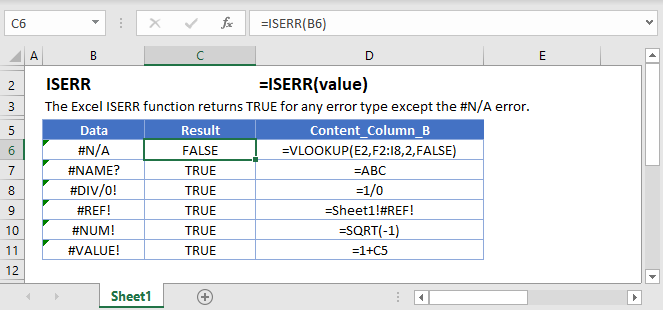## ISERR Function Overview

The ISERR Function Test if cell value is an error. Ignores #N/A. Returns TRUE or FALSE.

To use the ISERR Excel Worksheet Function, select a cell and type: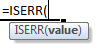(Notice how the formula inputs appear)

### ISERR Function Syntax and Inputs:

`=ISERR(VALUE)`

value – The test value

AutoMacro - VBA Code Generator

## How to use the ISERR Function

The ISERR Function checks if a calculation results in any error, except the #N/A error.

`=ISERR(A2)`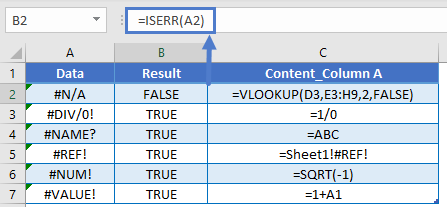### ISERROR, ISERR, and ISNA

There are two other error checking “is” functions: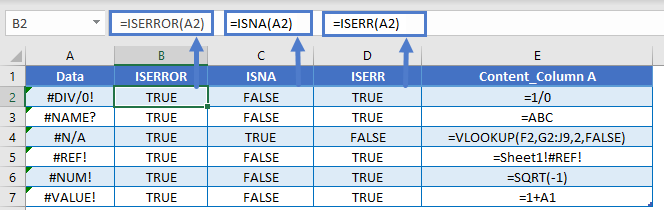There are also the IFERROR and IFNA Functions that test for errors, allowing you to do something if an error is found.

```=IFERROR(VLOOKUP(A2,\$E\$2:\$F\$7,2,FALSE),"No Data")
=IFNA(VLOOKUP(A2,\$E\$2:\$F\$7,2,FALSE),"No Data")```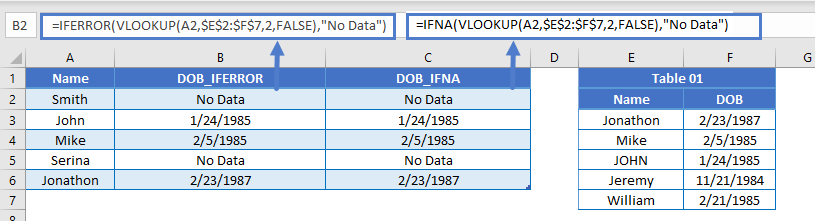### Other Logical Functions

Excel / Google Sheets contain many other logical functions to perform other logical tests. Here is a list:

IF / IS Functions
iferror
iserror
isna
iserr
isblank
isnumber
istext
isnontext
isformula
islogical
isref
iseven
isodd

## ISERR in Google Sheets

The ISERR Function works exactly the same in Google Sheets as in Excel: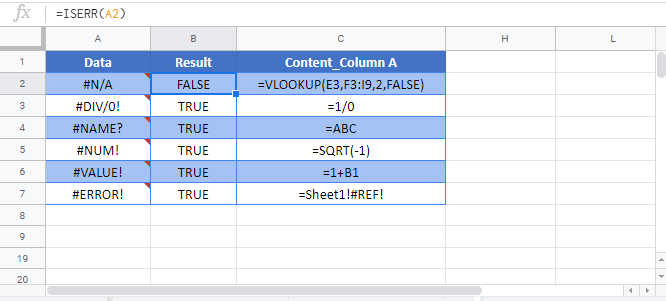## ISERR Examples in VBA

You can also use the ISERR function in VBA. Type:
`application.worksheetfunction.iserr(value)`
For the function arguments (value, etc.), you can either enter them directly into the function, or define variables to use instead.

Return to the List of all Functions in Excel

## Excel Practice Worksheet

Practice Excel functions and formulas with our 100% free practice worksheets!

• Automatically Graded Exercises
• Learn Excel, Inside Excel!